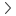# Margin Calculator

Traders' toolsMargin calculator

0

Synthetic

Financial

### How to calculate margin

The margin required for a contract on Deriv MT5 is calculated based on the formula:

Margin = (volume × contract size × asset price) ÷ leverage

This gives you the margin requirement in the quote currency for forex pairs, or in the denomination of the underlying asset for other instruments.

For instance, if you are trading the USD/CHF forex pair, the margin requirement will be calculated in Swiss Franc (CHF) which is the quote currency. On the other hand, if you are trading Volatility Index 75, then the margin requirement will be calculated in US Dollar (USD), which is the denomination of the underlying asset – Volatility Index 75.

### Example calculation

Let’s say you want to trade two lots of EUR/USD with an asset price of 1.10 USD and leverage of 100.

( 2
Volume
x
100,000
Contract size 1
x
1.10 )
Asset price
÷
100
Leverage
=
2,200
Margin required
( 2
Volume
x
100,000
Contract size 1
x
1.10 )
Asset price
÷
100
Leverage
=
2,200
Margin required
1. One standard lot of forex = 100,000 units

So you will require a margin rate of 2,200 USD to open the above position.

Note that these are approximate values only and will differ depending on the leverage that is set for your account and the asset you want to trade.